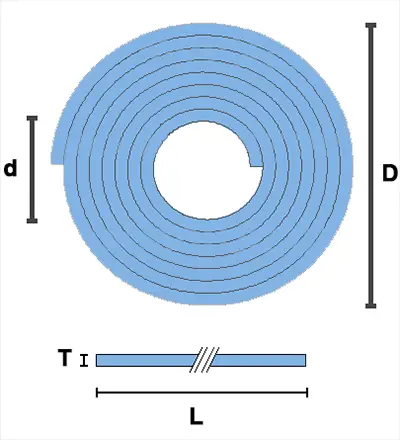# Roll Length Calculator

When you have material rolled up into a cylinder, you can swiftly and simply calculate its length using this roll length calculator.

How to use this calculator: Simply input measurements for the inner and outer dimensions of your roll of material and how thick the material is, and then click "Calculate".

Roll Length Calculator

Results

## Calculating the length of a roll

If you take a cross-section of a roll, it forms an annulus. If you lay the material flat, this cross-sectional shape will be a long thin rectangle. These two shapes will have equal areas, and this is the key to finding out the length of your roll.

Look at this image:Let's call the outside diameter of the roll of material D, the inner diameter d, the material's thickness T, and the length of the material L: all of these can then be factored into the following equation:

π × (D2 / 4 − d2 / 4) = L × T

On the left of the equation we have the annulus' area, and on the right we have the rectangle's area. To find L, we just have to divide each side by T:

π × (D2 / 4 − d2 / 4) / T = L

Where π is the constant (3.141592654).

The formula provides us with a length of any roll of material providing we have the thickness of the material and the inner and outer diameters of the cylinder. Make sure you use the same units for all inputs.

## Example

Imagine you have a cylinder material that has an outer diameter of 500 mm, an inner diameter of 100 mm, and that the material is 0.5 mm thick. We can input these figures into our equation as D = 500, d = 100, and T = 0.5, and we will thereby find the length:

π × (D2 / 4 − d2 / 4) / T = L

= π × (5002 / 4 − 1002 / 4) / 0.5

= 3.141592654 × (62500 − 2500) / 0.5

= 376991.112 mm = 376.991 meters, or 377 meters (rounded to 3 sig figs).